Courses

# Sound Waves (Part - 1) - Physics, Solution by DC Pandey NEET Notes | EduRev

## DC Pandey (Questions & Solutions) of Physics: NEET

Created by: Ciel Knowledge

## NEET : Sound Waves (Part - 1) - Physics, Solution by DC Pandey NEET Notes | EduRev

The document Sound Waves (Part - 1) - Physics, Solution by DC Pandey NEET Notes | EduRev is a part of the NEET Course DC Pandey (Questions & Solutions) of Physics: NEET.
All you need of NEET at this link: NEET

Introductory Exercise 16.1

Ques 1: Calculate the bulk modulus of air from the following data for a sound wave of wavelength 35 cm travelling in air. The pressure at a point varies between (105 ± 14) Pa and the particles of the air vibrate in SHM of amplitude 5.5 × 10-6 m.
Sol: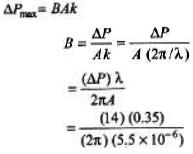= 1.4 × 105N/m2

Ques 2: Find the minimum and maximum wavelengths of sound in water that is in the audible range for an average human ear. Speed of sound in water is 1450 m/s.
Sol: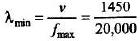= 0.0725 m = 7.25 cm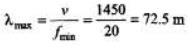Ques 3: A typical loud sound wave with a frequency of 1 kHz has a pressure amplitude of about 10 Pa (a) At t = 0, the pressure is a maximum at some point x1. What is the displacement at that point at t = 0?
(b) What is the maximum value of the displacement at any time and place ? Take the density of air to be 1.29 kg/m3 and speed of sound in air is 340 m/s.
Sol:
(a) Displacement is zero when pressure is maximum.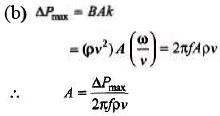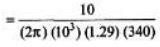= 3.63 × 10-6 m

Ques 4:  The pressure variat ion in a sound wave in air is given by
ΔP = 12 sin(8.18x - 2700t +π/4) N/m2
Find the displacement amplitude. Density of air = 1.29 kg/m3.
Sol:
In the above problem, we have found that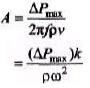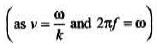Now substituting the value, we have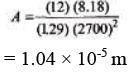Introductory Exercise 16.2

Ques 1: Calculate the temperature at which the velocity of sound in air is double its velocity at 0°C.
Sol:
v ∝ √T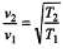or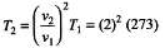= 1092 K = 819°C

Ques 2: Calculate the difference in the speeds of sound in air at -3°C, 60 cm pressure of mercury and 30°C, 75 cm pressure of mercury. The speed of sound in air at 0°C is 332 m/s.
Sol:
v ∝ √T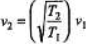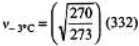= 330.17 m/s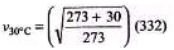= 349.77 m/s
The difference in these two speeds is approximately 20 m/s.

Ques 3: In a liquid wit h density 900 kg/m3, longitudinal waves with frequency 250 Hz are found to have wavelength 8.0 m. Calculate the bulk modulus of the liquid.
Sol: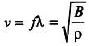∴ B = ρ (fλ)2
= (900) (250 × 8)2 = 3.6 × 109 N/m2

Ques 4: Calculate the speed of sound in oxygen at 273 K.
Sol: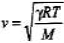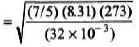= 315 m/s

Introductory Exercise 16.3

Ques 1: A sound wave in air has a frequency of 300 Hz and a displacement amplitude of 6.0 × 10 -3 mm. For this sound wave calculate the
(a) pressure amplitude
(b) intensity
(c) sound intensity level (in dB)
Speed of sound = 344 m/s and density of air = 1.2 kg/m3.

Sol: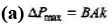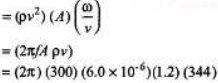= 4.67 Pa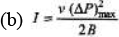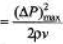(as  B= ρv2)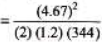= 0.0264 W/m2 = 2.64 × 10-2 W/m2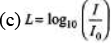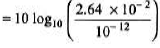= 104 dB

Ques 2: Most people interpret a 9.0 dB increase in sound intensity level as a doubling in loudness. By what factor must the sound intensity be increased to double the loudness?Sol: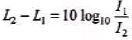Given L2 - L1 =9 dB
Solving the equation we get,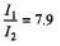Ques 3: A baby's mouth is 30 cm from her father's ear and 3.0 m from her mother's ear. What is the, difference between the sound intensity levels heard by the father and by the mother.
Sol: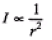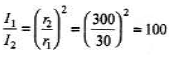Now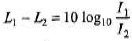SubstitutingWe get, Lx1 - L2 - 20 dB

Ques 4: The faintest sound that can be heard has a pressure amplitude of about 2 × 10-5 N/m2 and the loudest that can be heard without pain has a pressure amplitude of about 28 N/m2. Determine in each case
(a) the intensity of the sound both in W/mand in dB and
(b) the amplitude of the oscillations if the frequency is 500 Hz. Assume an air density of 1.29 kg/mand a velocity of sound is 345 m/s.
Sol: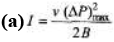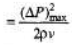For finest sound,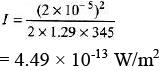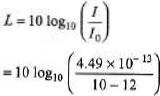= - 3.48 dB
Same formulae can be applied for loudest sound.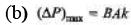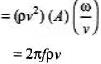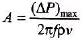For finest sound,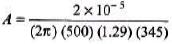= 1.43 × 10-11 m

Offer running on EduRev: Apply code STAYHOME200 to get INR 200 off on our premium plan EduRev Infinity!

210 docs

,

,

,

,

,

,

,

,

,

,

,

,

,

,

,

,

,

,

,

,

,

,

,

,

;